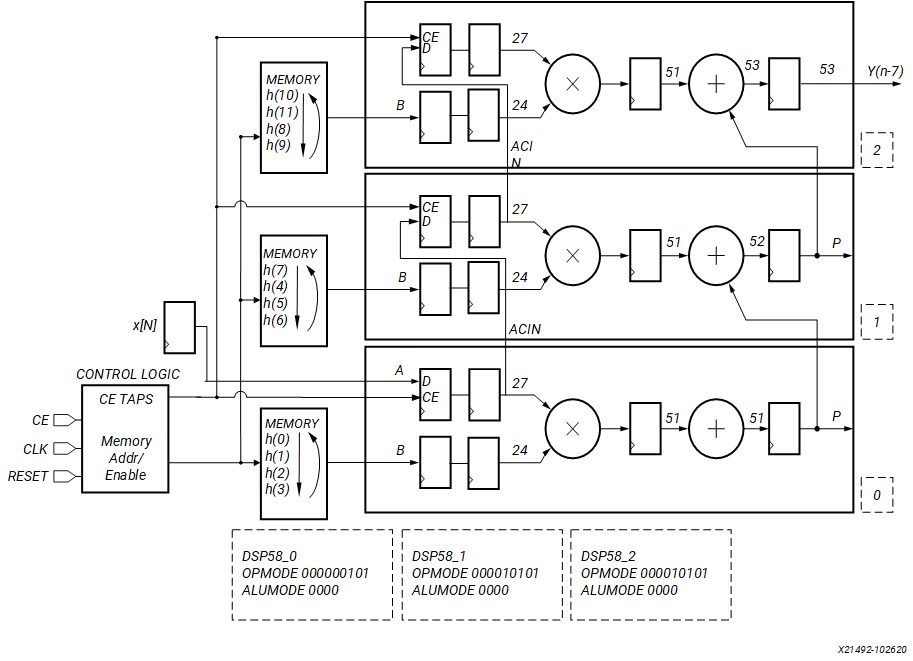# Interpolating

## Versal ACAP DSP Engine Architecture Manual (AM004)

Document ID
AM004
Release Date
2022-09-11
Revision
1.2.1 English

Increasing the number of samples representing a signal is called interpolating or up-sampling. Interpolation is used in applications like medical imaging and SDTV-to-HDTV conversions. A 12-tap 1:4 interpolator is illustrated in the following figure. A 1:4 interpolation results in four output samples for every one input sample. The 12 taps are the 12 coefficients that are used to calculate the four output samples. The following equations describe the relation between the input samples (x), coefficients (h), and the output samples (y).

y0 = (h0 × xn) + (h4 × xn – 1) + (h8 × xn – 2)
y1 = (h1 × xn) + (h5 × xn – 1) + (h9 × xn – 2)
y2 = (h2 × xn) + (h6 × xn – 1) + (h10 × xn – 2)
y3 = (h3 × xn) + (h7 × xn – 1) + (h11 × xn – 2)
Figure 1. 12-Tap 1:4 InterpolatorIn the interpolating FIR filter design, the phases are related to the number of DSP58s, and the number of coefficients is related to the memory depth (if RAM is used) or number of registers (SRL16). Referring to the figure above, the 12 coefficients are cycled through the DSP58s. These coefficients can be stored in shift registers (SRL16) or memories and grouped in each DSP58.

The reference design files associated with this use case are available in the multirate_FIR/polyphase_interpolating_FIR directory in the design archive file, am004-versal-dsp-engine.zip.